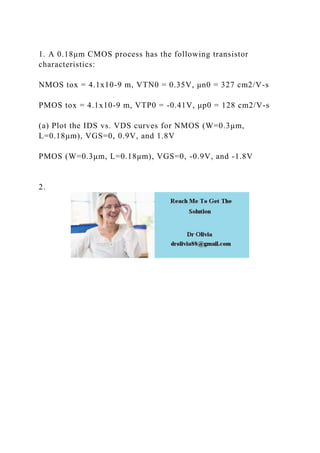Seu SlideShare está sendo baixado. ×

# 1. A 0.18μm CMOS process has the following transistor characteristic.docx

Anúncio
Anúncio
Anúncio
Anúncio
Anúncio
Anúncio
Anúncio
Anúncio
Anúncio
Anúncio
AnúncioCarregando em…3
×

1 de 1 Anúncio

# 1. A 0.18μm CMOS process has the following transistor characteristic.docx

1. A 0.18μm CMOS process has the following transistor characteristics:

NMOS tox = 4.1x10-9 m, VTN0 = 0.35V, μn0 = 327 cm2/V-s

PMOS tox = 4.1x10-9 m, VTP0 = -0.41V, μp0 = 128 cm2/V-s

(a) Plot the IDS vs. VDS curves for NMOS (W=0.3μm, L=0.18μm), VGS=0, 0.9V, and 1.8V

PMOS (W=0.3μm, L=0.18μm), VGS=0, -0.9V, and -1.8V

2.
.

1. A 0.18μm CMOS process has the following transistor characteristics:

NMOS tox = 4.1x10-9 m, VTN0 = 0.35V, μn0 = 327 cm2/V-s

PMOS tox = 4.1x10-9 m, VTP0 = -0.41V, μp0 = 128 cm2/V-s

(a) Plot the IDS vs. VDS curves for NMOS (W=0.3μm, L=0.18μm), VGS=0, 0.9V, and 1.8V

PMOS (W=0.3μm, L=0.18μm), VGS=0, -0.9V, and -1.8V

2.
.

Anúncio
Anúncio

### 1. A 0.18μm CMOS process has the following transistor characteristic.docx

1. 1. 1. A 0.18μm CMOS process has the following transistor characteristics: NMOS tox = 4.1x10-9 m, VTN0 = 0.35V, μn0 = 327 cm2/V-s PMOS tox = 4.1x10-9 m, VTP0 = -0.41V, μp0 = 128 cm2/V-s (a) Plot the IDS vs. VDS curves for NMOS (W=0.3μm, L=0.18μm), VGS=0, 0.9V, and 1.8V PMOS (W=0.3μm, L=0.18μm), VGS=0, -0.9V, and -1.8V 2.